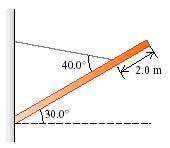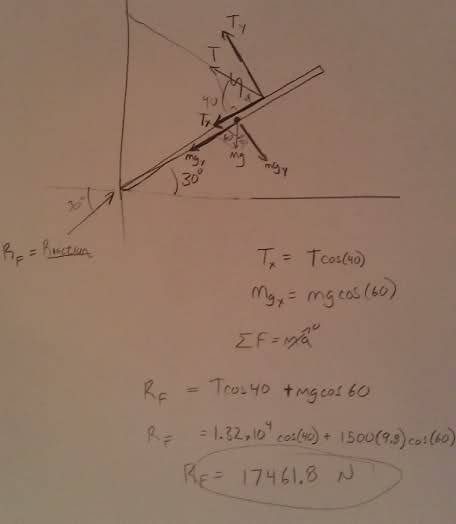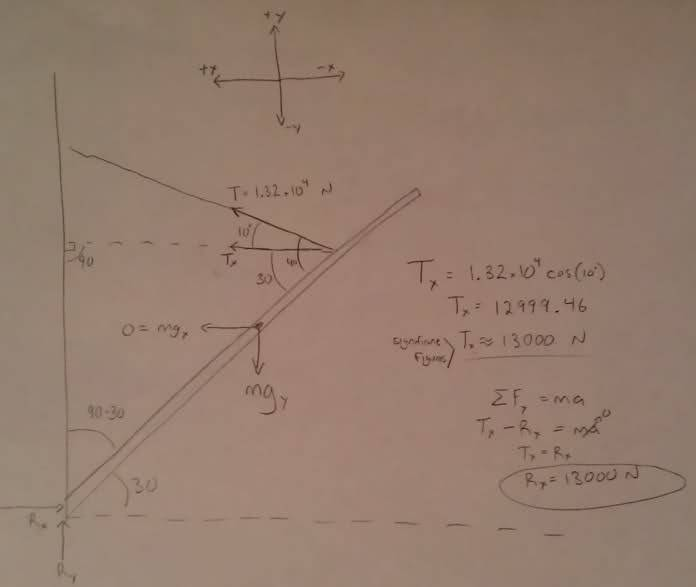# Force of Bar on wall

## Homework Statement

How hard does the beam push inward on the wall?## The Attempt at a Solution

I know that the tension on the cable is 1.32*10^4 N

From there I turned the weight and tension into x/y components and tried to add the x components, so that:
1.32*10^4cos(40) + 14700sin(30) = 17461.79 N

I don't understand why this is not correct?

Last edited:

PhanthomJay
Homework Helper
Gold Member
what is the weight and length of the bar?

Sorry, the bar is 8m long, and 1500kg.

PhanthomJay
Homework Helper
Gold Member
There are no z components, only x an y components. The weight has a y component only. The tension force has x and y components. The force of the wall on the bar has x and y components. Use Newton 1 in the x and y directions to solve for the x and y components of the wall force on the bar. Your tension force is correct.

Thanks for taking the time to respond Jay,

That z component was a typo, I meant to say x-component.

I believe I did what you described above. I got the reaction force as shown in this image:
(increasing x is down an left)What exactly am I doing incorrectly?

PhanthomJay
Homework Helper
Gold Member
The reaction force at the wall is not directed parallel to the bar...that only occurs for 2 force members , like the cable. You'll have to include the componets perpendicular to the bar also. You also do not have to break up the components into forces parallel and perpendicular to the incline, although that is acceptable. But you can also look at just x and y (horiz and vert) components.

gneill
Mentor
The bar presses on the wall at an angle (30° to the horizontal). Some of the force is directed downward (pushing the wall down into the ground), some is directed "inward".

Like this?This solution of 13000 N is also incorrect.

I'm not sure what you mean by breaking it up into components perpendicular to the bar. I didn't think that I could use torque to find the force for this question.

Alternatively, 17461.8cos(30), my original reaction force that was angled to the wall, resolved into it's x component, produces 15122.36?

PhanthomJay
Homework Helper
Gold Member
Like this?This solution of 13000 N is also incorrect.
that's the x component.. Now look in the y direction to get the y component.
I'm not sure what you mean by breaking it up into components perpendicular to the bar.
that is what you did in your post 5. It is not necessary to do it this way.
Alternatively, 17461.8cos(30), my original reaction force that was angled to the wall, resolved into it's x component, produces 15122.36?
No, that is the wrong way to do it. Your x component is now corrrect; solve for the y component in a similar manner using newton 1.

Okay, so:
$$mg-R_{y}-Tsin(80)=0$$
$$R_{y} =mg-Tsin(80)$$
$$R_{y} =1500(9.8)-(1.32*10^{4})sin(80)$$
$$R_{y} = 1700.54$$

So now my Y component is: 1700 N, and my x is 13000 N. Do they want the sum of the two for this "inward" force from the bar on the wall? It's not simply the x component as I initially thought.

Thanks.

PhanthomJay
Homework Helper
Gold Member
That should be T sin 10 for the vert comp of the tension force. Then once you correctly solve for Ry, you have to add them up vectorially (not algebraically) to get the total force at the wall, using Pythagoras.

I get 17970.9 N from the vector sum of the two. Does that seem right? Now I need to go back and re-analyze how I am approaching these problems. I have no problems with torque and such, but this one, idk what my problem is. Thank you all for the help.

PhanthomJay